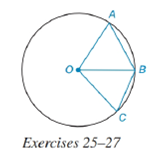Chapter 6.4, Problem 23E### Elementary Geometry for College St...

6th Edition
Daniel C. Alexander + 1 other
ISBN: 9781285195698

#### Solutions

Chapter
Section### Elementary Geometry for College St...

6th Edition
Daniel C. Alexander + 1 other
ISBN: 9781285195698
Textbook Problem
1 views

# In circle O , points A , B , and C are on the circle such that m A B ⌢ = 60 ° and m B C ⌢ = 40 ° .a) How are m ∠ A O B and m ∠ B O C related?b) How are A B and B C related?To determine

(a)

To find:

The relation between mAOB and mBOC.

Explanation

Given:

Circle O and points A, B, and C are on the circle such that mAB=60° and mBC=40°. The figure given below

Theorem Used:

In a circle (or in congruent circles) containing two unequal arcs, the larger arc corresponds to the larger central angle.

Calculation:

Using the theorem:

In a circle (or in congruent circles) containing two unequal arcs, the larger arc corresponds to the larger central angle.

Since the arcs mAB=60° and mBC=40°

To determine

(b)

To find:

The relation between AB and BC.

### Still sussing out bartleby?

Check out a sample textbook solution.

See a sample solution

#### The Solution to Your Study Problems

Bartleby provides explanations to thousands of textbook problems written by our experts, many with advanced degrees!

Get Started

#### Find more solutions based on key concepts